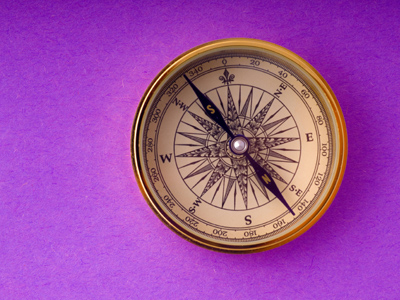The compass point opposite South East is North West.

# Position 2

This Math quiz is called 'Position 2' and it has been written by teachers to help you if you are studying the subject at elementary school. Playing educational quizzes is an enjoyable way to learn if you are in the 3rd, 4th or 5th grade - aged 8 to 11.

It costs only \$12.50 per month to play this quiz and over 3,500 others that help you with your school work. You can subscribe on the page at Join Us

Working out position usually involves tools such as compasses, used to show the direction of North, or protractors, which are used to measure the number of degrees in angles. There are 360 degrees in circle and 180 degrees in a half circle. 180 degrees are not only found in a half circle - a triangle and a straight line also both have 180 degrees.

1.
If a clock hand moves from 6 to 12 how many degrees has it turned?
30o
180o
90o
45o
From 6 to 12 is equivalent to one half turn
2.
Where is the compass point North East found?
Between North and South
Between North and West
Between North and East
Between South and East
On a clock face North East would be halfway between the 1 and the 2
3.
How many degrees is a right angle turn?
180o
30o
45o
90o
A right angle turn is the same as a quarter turn
4.
What is the compass point between South and West?
South West
South East
South North
North West
To remember the position of the compass points, use a rhyme such as 'Never Eat Slimy Worms'
5.
Which compass point is between South and East?
South East
South West
North East
South North
On a clock face South East would be halfway between the 4 and the 5
6.
How many degrees is one whole turn?
180o
360o
90o
400o
Half a turn is 180o
7.
Which compass point is opposite South East?
North East
South West
North West
South
On a clock face North West would be halfway between the 10 and the 11
8.
How many degrees in a quarter turn?
180o
360o
45o
90o
One quarter turn will be equivalent to one quarter of 360o
9.
If a clock hand moves from 12 to 3 how much has it turned?
One quarter
Half
Three quarters
One whole
When the minute hand points to the 3 on a clock face it shows one quarter of an hour
10.
If a clock hand moves from 12 to 1 how many degrees has it turned?
180o
30o
90o
45o
There are 12 hours on a clock face so to work out how many degrees one hour is, divide 360 by 12
Author:  Amanda Swift